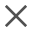Basic Knowledge | 2019.06.28 | 4592
Thermal transmittance and Thermal Conductivity
In short, the “thermal conductivity” represents the properties of the materials unrelated to the actual thickness of the plate or the surrounding environment while the “Thermal transmittance (U-value)” is related to both the thickness of the plate and its surrounding environment.

Thermal transmittance (known as “U value”), refers to the amount of heat passing through an one square meter area in one second under the stable heat transfer condition with the air temperature difference between the two side of an enclosure equal to 1 degree (K, ℃). The Thermal transmittance of building glass refers to the amount of heat transferred from the air of one side of the glass assembly to the air of the other side in a unit area per unit time when the temperature difference between the two sides equal to 1K (℃) .

The metric unit of the Thermal transmittance is W/(㎡·K), where W is the thermal power, ㎡ is the area of the glass, and K is the Celsius temperature. The imperial unit of the heat transfer coefficient is Btu/(ft2·h·℉), where Btu is the British thermal unit of heat, h is the hour, ft2 is the square feet, and ℉ is the temperature in Fahrenheit. The conversion relationship between the two units is as follows: 1Btu/(ft2·h·℉) =5.678W/(㎡·K). China and major European countries adopt the metric system while the United States and other North American countries use the Imperial system.

Thermal conductivity refers to the amount of heat conducted through one square meter area of a 1m thick material under stable thermal conditions with the temperature difference between the two surfaces is 1 degree (K，°C). It is commonly denoted by λ. The unit is W/m·k (here, K can be substituted with ℃). The thermal conductivity is related to the composition, density, moisture content, temperature and other factors of the material. The size, shape, and thickness of the material are not relevant. As it is determined by the composition of the material itself, the coefficient of thermal conductivity of the same material is the same.

In short, the “thermal conductivity” represents the properties of the materials unrelated to the actual thickness of the plate or the surrounding environment while the “Thermal transmittance (U-value)” is related to both the thickness of the plate and its surrounding environment.